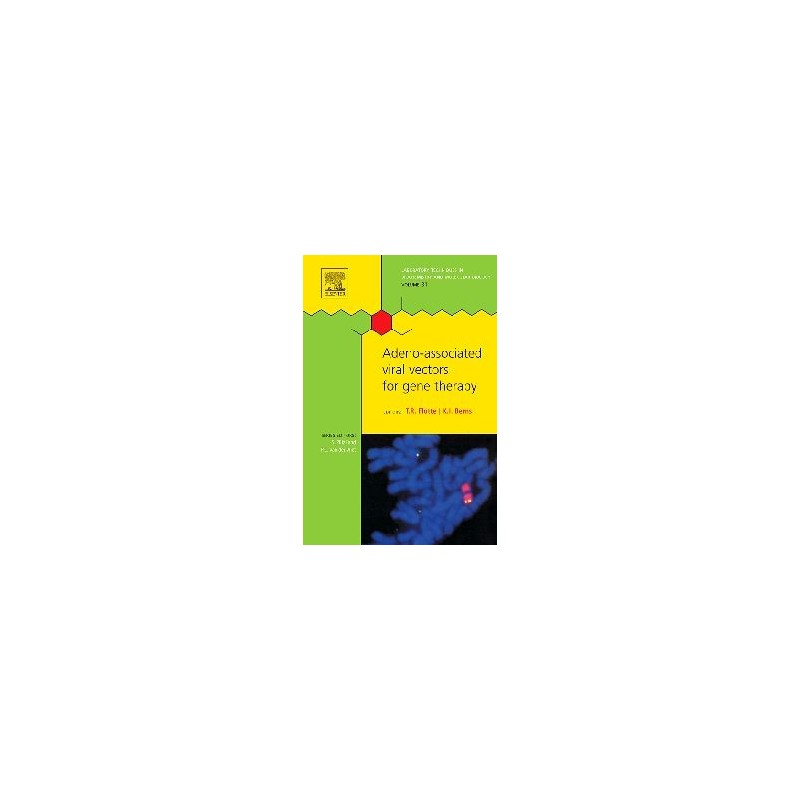﻿Zobacz większe

# Introduction to Probability and Statistics for Engineers and Scientists

Sheldon Ross

ID: 173268

### Więcej informacji

This updated text provides a superior introduction to applied probability and statistics for engineering or science majors. Ross emphasizes the manner in which probability yields insight into statistical problems; ultimately resulting in an intuitive understanding of the statistical procedures most often used by practicing engineers and scientists. Real data sets are incorporated in a wide variety of exercises and examples throughout the book, and this emphasis on data motivates the probability coverage.

As with the previous editions, Ross' text has remendously clear exposition, plus real-data examples and exercises throughout the text. Numerous exercises, examples, and applications
apply probability theory to everyday statistical problems and situations.

Also Available: Student Solutions Manual for Introduction to Probability and Statistics for Engineers and Scientists, 4e ISBN:9780123705280

New to the 4th Edition:
- New Chapter on Simulation, Bootstrap Statistical Methods, and Permutation Tests
- 20% New Updated problem sets and applications, that demonstrate updated applications to engineering as well as biological, physical and computer science
- New Real data examples that use significant real data from actual studies across life science, engineering, computing and business
- New End of Chapter review material that emphasizes key ideas as well as the risks associated with practical application of the material

Preface
Ch1 Introduction to Statistics
Ch2 Descriptive Statistics
Ch3 Elements of Probability
Ch4 Random Variables and Expectation
Ch5 Special Random Variables
Ch6 Distributions of Sampling Statistics
Ch7 Parameter Estimation
Ch8 Hypothesis Testing
Ch9 Regression
Ch10 Analysis of Variance
Ch11 Goodness of Fit Tests and Categorical Data Analysis
Ch12 NonparametricHypothesisTests
Ch13 Quality Control
Ch14 LifeTesting
Ch15 Simulation, Bootstrap Statistical Methods, and Permutation Tests
Appendix of Tables